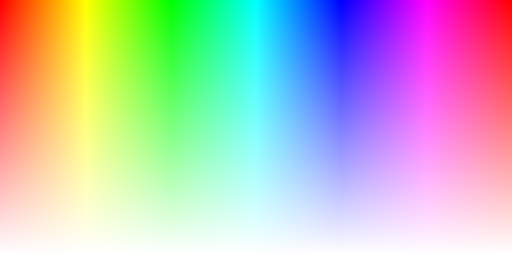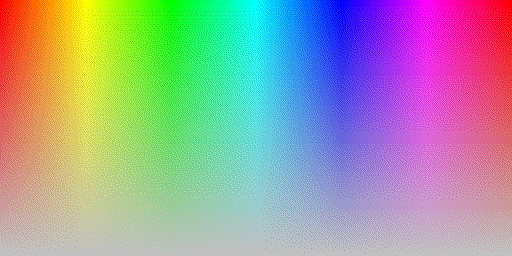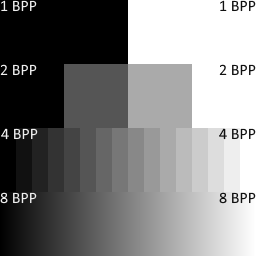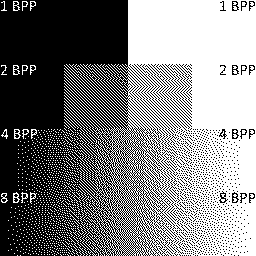﻿ErrorDiffusionDitherer Constructor

# ErrorDiffusionDitherer Constructor

Initializes a new instance of the ErrorDiffusionDitherer class using the specified matrix, divisor and matrixFirstPixelIndex.

## Definition

Namespace: KGySoft.Drawing.Imaging
Assembly: KGySoft.Drawing.Core (in KGySoft.Drawing.Core.dll) Version: 7.2.0
C#
``````public ErrorDiffusionDitherer(
byte[,] matrix,
int divisor,
int matrixFirstPixelIndex,
bool serpentineProcessing = false,
bool? byBrightness = null
)``````

#### Parameters

matrix  Byte
A matrix to be used as the coefficients for the quantization errors to be propagated to the neighboring pixels.
divisor  Int32
Each elements in the matrix will be divided by this value. If less than the sum of the elements in the matrix, then only a fraction of the error will be propagated.
matrixFirstPixelIndex  Int32
Specifies the first effective index in the first row of the matrix. If larger than zero, then the error will be propagated also to the bottom-left direction. Must be between 0 and matrix width, excluding upper bound.
serpentineProcessing  Boolean  (Optional)
to process odd lines right-to-left and even lines left-to-right; to process all lines left-to-right.
See the Remarks section of the ConfigureProcessingDirection method for details. This parameter is optional.
Default value: .
byBrightness  NullableBoolean  (Optional)
to apply the same quantization error on every color channel determined by brightness difference; to handle quantization errors on each color channels independently; to auto select strategy. Deciding by brightness can produce a better result when fully saturated colors are mapped to a grayscale palette.
See the Remarks section of the ConfigureErrorDiffusionMode method for details. This parameter is optional.
Default value: .

## Example

The following example demonstrates how to use a custom ditherer using the ErrorDiffusionDitherer constructor:
C#
``````public static IReadWriteBitmapData ToCustomDithered(IReadWriteBitmapData source, IQuantizer quantizer)
{
// This is actually the Fan dithering (by Zhihang Fan), and uses the same coefficients
// as the Floyd-Steinberg dithering in a slightly different arrangement:
byte[,] matrix =
{
{ 0, 0, 0, 7 },
{ 1, 3, 5, 0 },
};

// The matrix values will be divided by this value to determine the portion
// of the quantization error to propagate to neighboring pixels:
int divisor = 16;

// The current pixel to be processed is always one pixel left from this index.
// This also means that if larger than 1, then some error is propagated also towards the
// (bottom-)left direction. For the matrix above value "3" means that whenever a pixel is
// processed, 7/16 of the error is propagated to the right, 1/16 and 3/16 to the
// bottom-left direction and 5/16 one pixel down from the current pixel.
int firstPixelIndex = 3;

IDitherer ditherer = new ErrorDiffusionDitherer(matrix, divisor, firstPixelIndex);

// a.) this solution returns a new bitmap data and does not change the original one:
return source.Clone(quantizer.PixelFormatHint, quantizer, ditherer);

// b.) alternatively, you can perform the dithering directly on the source bitmap data:
source.Dither(quantizer, ditherer);
return source;
}``````

The example above may produce the following results:
Original image
Quantized and dithered imageQuantizing with system default 8 BPP palette